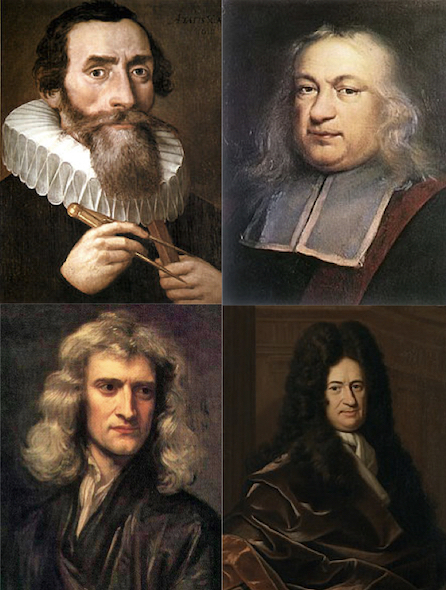1. Nothing

## Performance Optimization1#

Taylor series had been used for analyzing the performance surface and locating the optimum points of a certain performance index. This short post is a brief introduction to performance optimization and the following posts are the samples of three optimization algorithms categories:

Recall the analysis of the performance index, which is a function of the parameters of the model. Most of the optimization problems could not be solved analytically. So, searching for the whole solution space is a straightforward strategy. However, no algorithms or computers can search a whole parameter space even which has only 1 dimension to locate the optimal points of the surface. So we need a map.

These algorithms we discussed here are developed hundreds of years ago. And the basic principles of optimization were discovered during the $$17^{\text{th}}$$ century. And some of them were brought up by Kepler, Fermat, Newton, and Leibniz. However, computers are more powerful than paper and pencils. So, these optimization algorithms had been rediscovered and became a major branch of mathematics. Thanks to the brilliant scientists for their contribution to our human beings:All the algorithms we are going to talk about are iterative. Their general framework is:

1. start from some initial guess $$\mathbf{x}_0$$
2. update our guess in stages:
• $$\mathbf{x}_{k+1}=\mathbf{x}_k+\alpha_k \mathbf{p}_k$$
• or $$\Delta \mathbf{x}_k=\mathbf{x}_{k+1}-\mathbf{x}_k=\alpha_k \mathbf{p}_k$$
1. check the terminational condition, decide to go back to step 2 or to terminate the algorithm.

In the algorithms, $$\mathbf{p}_k$$ is the search direction that works like the compass of Captain Jack Sparrow. It can lead you to what you want. $$\alpha_k$$ is the step length which means how far we should go along the current direction of $$\mathbf{p}_k$$. $$\mathbf{x}_0$$ is an initial position of the algorithm.

These three elements are the basis of three categories of optimization algorithms. How to decide the direction we are going to search, how far we should go in a certain direction, and how to initiate the first point gave us research aspects.

1. Demuth, H.B., Beale, M.H., De Jess, O. and Hagan, M.T., 2014. Neural network design. Martin Hagan.↩︎